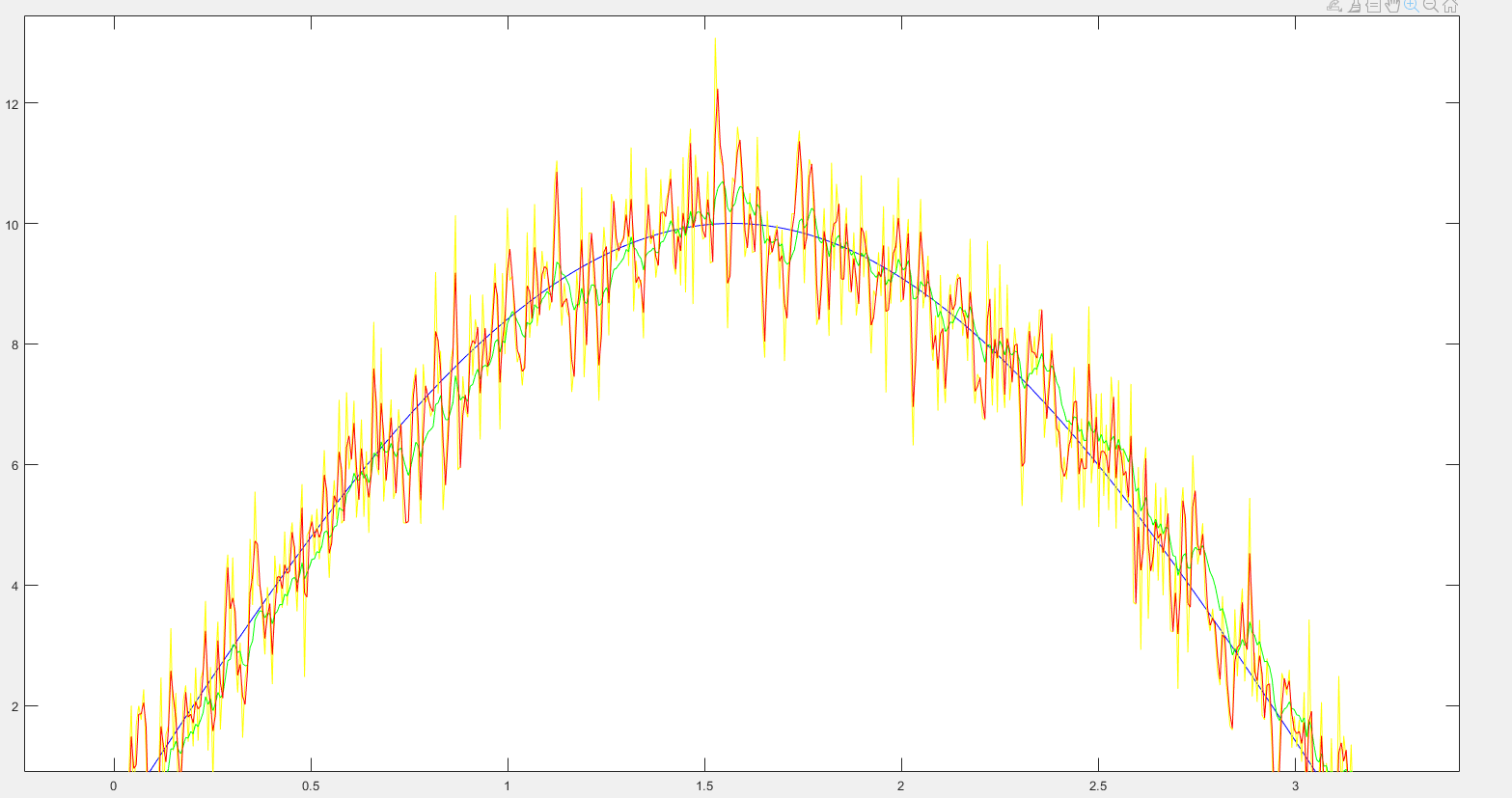# 基本动态系统模型

：是过程噪声，并假定其符合均值为零，协方差矩阵为  的多元正态分布。

：是观测噪声，其均值为零，协方差矩阵为  ,且服从正态分布。

在下面公式中会使用到

# 卡尔曼滤波器的公式

## 预测

：k-1时刻的最优估计值。

：k-1时刻在k时刻的最优估计值。

：k-1时刻估计值的协方差矩阵。

：k-1时刻在k时刻估计值的协方差矩阵。

：作用在上的状态变换模型。

：作用在控制器向量上的输入控制模型。

：控制向量。

：过程噪声的协方差矩阵。

## 更新

：测量残差

：测量残差协方差

：最优卡尔曼增益

：k时刻的最优估计值。

：k时刻估计值的协方差矩阵。

：观测噪声的协方差矩阵。

：观测模型，将真实空间状态映射成观测空间

：k时刻，真实状态的一个测量值

## 参数选定

：输入量。

：输入量的估计值。中间过程计算值。

：状态转移矩阵。

（1） 在标量卡尔曼滤波中，比如测量值是温度、湿度，一般认为下一个时刻该温度或者湿度维持不变，这种情况下状态转移矩阵通常就是标量1。

（2） 在导航和目标跟踪中卡尔曼滤波常被用来做位置估计，比如匀速直线运动（CV）和匀加速直线运动（CA）。

：表示可选的控制输入的增益，在大多数实际情况下并没有控制增益。

：表示k-1时刻的控制增益，一般没有这个变量，可以设为0。

：上一次滤波估计值协方差矩阵结果

：滤波估计值协方差矩阵中间值

：表示过程激励噪声的协方差，它是状态转移矩阵与实际过程之间的误差。在一阶的滤波中可认为是一个可调的参数。

：更新过程中的中间量

：本次滤波的结果，也是下一次滤波的输入值

：估计值协方差矩阵的输出值，也是下次的输入值。

：测量值，做输入值使用。

：观测噪声的协方差矩阵，此值一般取绝于测量器件的固有噪声。

：将观测值的位数与估计值的位数相匹配。一般在矩阵中的元素只有1和0。在一维中H=1

：单位矩阵。

# 卡尔曼滤波器的代码

,,,,,这些值取定值。

``````P_now = P_last + Q_cov;
K = P_now / (P_now + R_cov);
x_last = x_last + K * (z - x_last);
P_last = (1 - K) * P_now;``````

``````typedef struct Kalman_Filter{
float x_last;
float P_now;
float P_last;
float K;
float R_cov;
float Q_cov;
}KF_Struct; ``````

``````void KF_Struct_Init(KF_Struct* KFS)
{
KFS->x_last	=0;
KFS->P_now	=0;
KFS->P_last	=0.02;
KFS->K		=0;
KFS->Q_cov	=0.005;//过程激励噪声协方差,参数可调
KFS->R_cov	=0.5;//测量噪声协方差，与仪器测量的性质有关，参数可调
KFS->out	=0;
}``````

``````usage example：
KF_Struct KFS1;
KF_Struct_Init(&KFS1);``````

``````/*
* @brief    卡尔曼滤波器
* @param    KFS:卡尔曼滤波器结构体指针
* @param    z:测量仪器的输入量
* @return   当前时刻的最优估计值
*/
float KMFilter(KF_Struct* KFS,float z)
{
KFS->P_now = KFS->P_last + KFS->Q_cov;
KFS->K = KFS->P_now / (KFS->P_now + KFS->R_cov );
KFS->x_out = KFS->x_out + KFS->K * (z - KFS->x_out);
P_last = (1f - K)* P_now;

return KFS->x_out;
}``````

# 测试

``````N=500;
%kalman init
P_last = 0.02;
P_now = 0.0;
Q_cov = 0.013; %过程激励噪声协方差,参数可调
R_cov = 0.543; %测量噪声协方差，与仪器测量的性质有关，参数可调
x_last = 0.0;
K = 0.0;
out = 0.0;
%standard&bias
x = 0:pi/N:2*pi;
y = 10*sin(x);
%y=0;
bias = randn(1,2*N+1);
y_bias = y+bias;

%%%%%kalman
x_last = y_bias(1);
out(1)=0;
for i=2:2*N+1
z=y_bias(i);

P_now = P_last + Q_cov;
K = P_now / (P_now + R_cov );
x_last = x_last + K * (z - x_last);
P_last = (1 - K)* P_now;
out(i) = x_last;
end

%%%%一阶低通滤波
k=0.565;
ditong_filter(1)=0;
for j=2:2*N+1
ditong_filter(j)=k*y_bias(j)+(1-k)*y_bias(j-1);
end

plot(x,y,x,y_bias,x,out,x,ditong_filter);``````

实现的方式为在曲线上叠加一个随机噪声服从正态分布，然后通过滤波器进行滤波处理。下图是上图局部放大后的样子下图为初始值为恒定值的滤波曲线，图例与上图相同